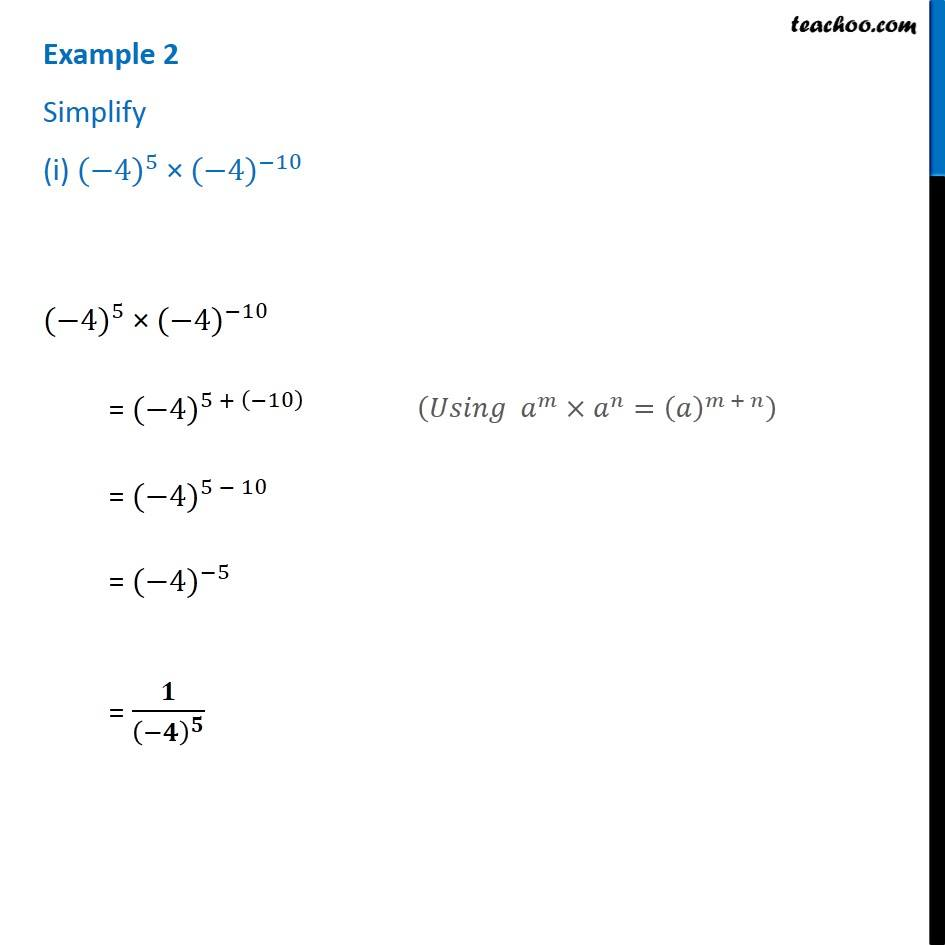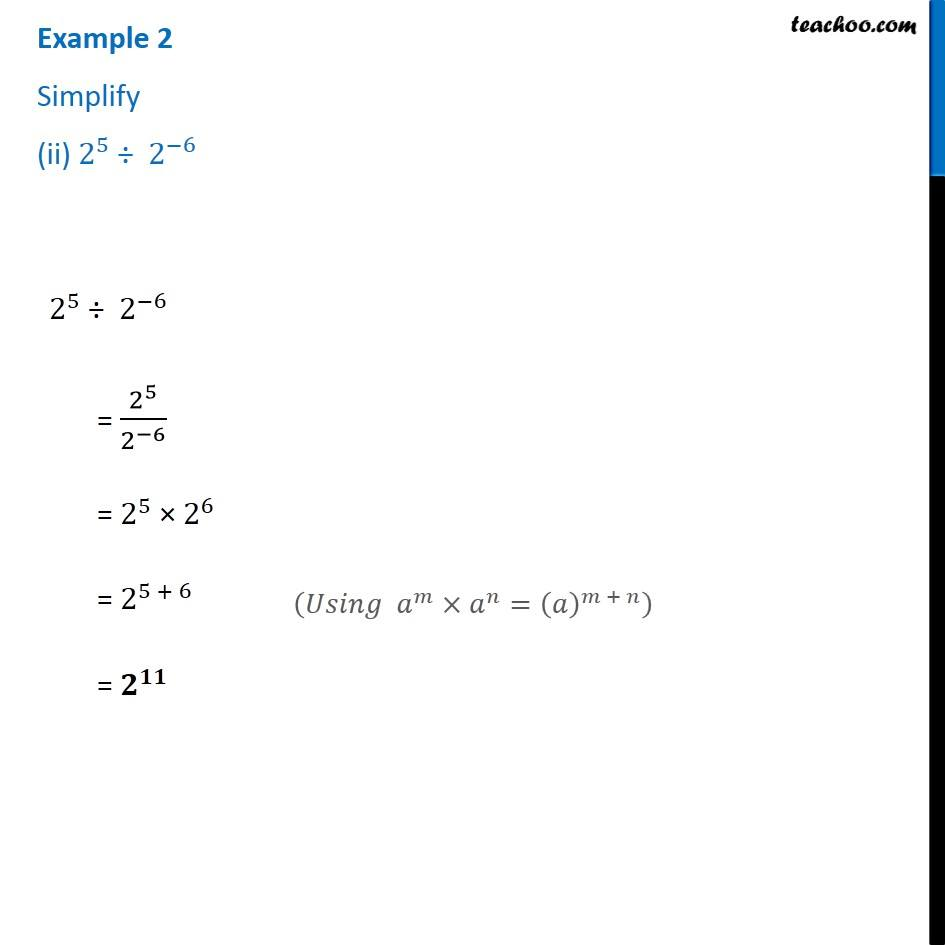1. Chapter 12 Class 8 Exponents and Powers
2. Serial order wise
3. Examples

Transcript

Example 2 Simplify (i) 〖(−4)〗^5 × 〖(−4)〗^(−10) 〖(−4)〗^5 × 〖(−4)〗^(−10) = 〖(−4)〗^(5 + (−10) ) = 〖(−4)〗^(5 − 10) = 〖(−4)〗^(−5) = 𝟏/(−𝟒)^𝟓 (𝑈𝑠𝑖𝑛𝑔 𝑎^𝑚×𝑎^𝑛=(𝑎)^(𝑚 + 𝑛) ) Example 2 Simplify (ii) 2^5 ÷ 2^(−6) 2^5 ÷ 2^(−6) = 2^5/2^(−6) = 2^5 × 2^6 = 2^(5 + 6) = 𝟐^𝟏𝟏 (𝑈𝑠𝑖𝑛𝑔 𝑎^𝑚×𝑎^𝑛=(𝑎)^(𝑚 + 𝑛) )

Examples